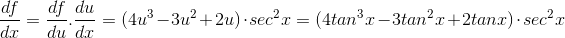High School Math : Finding First and Second Derivatives

Example Questions

Example Question #1 : Derivative Review

Find the second derivative of f(x).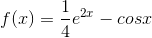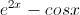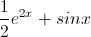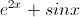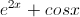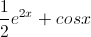Explanation:

First we should find the first derivative of. Remember the derivative of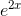is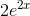and the derivative of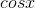is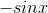: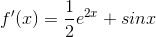The second derivative is just the derivative of the first derivative: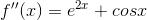Example Question #21 : Calculus Ii — Integrals

Find the derivative of the function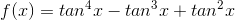.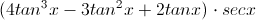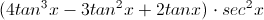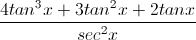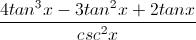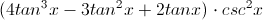Explanation:

We can use the Chain Rule:

Let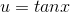, so that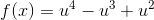.